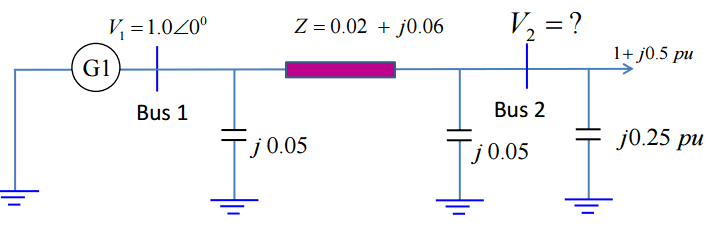# Power supplied by capacitor bank

< Mentor Note -- thread moved to HH from the technical forums, so no HH Template is shown >

Hi,

I have the following circuit, where V2 is found to be 0.964∠-3.3 (degrees) p.u.

Then I am to find the reactive power supplied by the capacitor bank (j0.25 p.u, to the right). I got the right number, but with wrong sign, could anyone parhaps tell me if my proposal at the end here is correct?

Calculations:

Current down the capacitor branch:
$I_{c} = V_{2} \times Y_{c} = (0.964 \angle-3.3) \times j0.25 = 0.241 \angle 86.7 p.u.\\ S = V \times I^{*} \rightarrow S_{c} = V_{2} \times I_{c}^{*} = 0.964 \angle(-3.3) \times 0.241 \angle(-86.7) = -j0.2323 p.u.$

So I get that Q = -0.2323, however it should have been positive since the bank is supplying the bus with power, and since injected power into a bus is defined positive.

So then I guess that current is defined as positive when entering the bus, and negative when leaving, and because of that I_c should be calculated like this instead:

$I_{c} = (0 - V_{2}) \times Y_{c}$

Thanks for any feedback.

Best regardsLast edited by a moderator:

Is it really the reactance of capacitor?
I think there is mistake in question
the reactance of capacitor is always given by (-j/wC) where w is angular frequqncy....If we solve the value for C, we get a negative value.....
So, I think there is mistake in question

Is it really the reactance of capacitor?
I think there is mistake in question
the reactance of capacitor is always given by (-j/wC) where w is angular frequqncy....If we solve the value for C, we get a negative value.....
So, I think there is mistake in question

j0.25 is the admittance not impedance, so if I solve the value for C, I get a positive value.

$Y = \frac{1}{Z} \rightarrow Z = \frac{1}{Y} = \frac{1}{j0.25} = -j4 \\ Z = \frac{1}{j\omega C} = -j4 \rightarrow C = \frac{1}{4 \times \omega}$

I did not write that it was the admittance, I just wrote Y instead of Z in the equations, I should have been more clear.

gneill
Mentor
When you calculated the current for the capacitor bank, what direction was the assumed current?

If the the bank is supplying power, what direction should the assumed current be?

When I calculated and got the wrong sign I did the following:

$(V_2-V_{Ground}) \times Y_c$
When I do it like this I am assuming the current leaves the bus, which should not be the case, therefore I should do it like this:
$(V_{Ground}-V_2) \times Y_c$
Doing it this way assumes that current flows into the bus.

Is my way of thinking OK? I'm new to "electrical stuff", and therefore I sometimes fail at stuff like this.

gneill
Mentor
When I calculated and got the wrong sign I did the following:

$(V_2-V_{Ground}) \times Y_c$
When I do it like this I am assuming the current leaves the bus, which should not be the case, therefore I should do it like this:
$(V_{Ground}-V_2) \times Y_c$
Doing it this way assumes that current flows into the bus.

Is my way of thinking OK? I'm new to "electrical stuff", and therefore I sometimes fail at stuff like this.
Yes, that would be the right way to look at it.

I think you should look at the definition and formula of S(reactive power) for clarity.